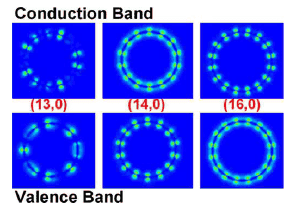# Band decomposed charge density

• Douasing

#### Douasing

Hi,there are many literatures concered with band decomposed charge density(As an example,see FIG.3 in PHYSICAL REVIEW B 78, 235430 2008).
For condution band,there should not be charges or electrons when the system is in the ground state,but,those literares still show a band decomposed charge density picture,I'm very confused with that.
Douasing

Of course. The link is http://journals.aps.org/prb/pdf/10.1103/PhysRevB.78.235430 [Broken]
And the FiG.3 is shown as follows:FIG. 3. Color online Band decomposed charge density at the Gamma
point for 13,0, 14,0, and 16,0.

Last edited by a moderator:
In general, the conduction band isn't empty, not even in the ground state, especially in metals. So I don't see a reason as why not to calculate charge density. The pictures you are showing is the electron density $$|\psi|^2$$ as would result from a single Bloch function or orbital being filled. Obviously, you can plot it irrespective of whether the orbital is filled or not.

In general, the conduction band isn't empty, not even in the ground state, especially in metals. So I don't see a reason as why not to calculate charge density. The pictures you are showing is the electron density $$|\psi|^2$$ as would result from a single Bloch function or orbital being filled. Obviously, you can plot it irrespective of whether the orbital is filled or not.
In VASP, there is a parameter called NBMOD as follows:
NBMOD=0 : Take all bands to calculate "the charge density", even unoccupied bands are taken into account.
NBMOD=-1: Calculate the "total charge density" as usual. This is the default value if nothing else is given.
What's the difference bewteen "the charge density" and "the total charge density" ?